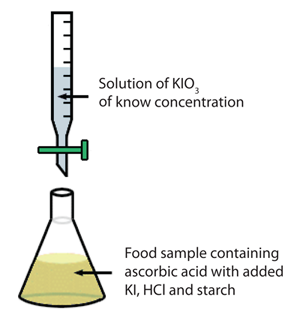# Colorimetric Titration Experiment - Determine the amount of vitamin C in a medium peach

A suitable method for the determination of vitamin C (ascorbic acid, C6H8O6) is a titration with potassium iodate (KIO3). In this reaction, potassium iodate is used as a titrant and when added to an ascorbic acid solution that contains strong acid and potassium iodide (KI), the potassium iodate reacts with potassium iodide, liberating molecular iodine (I2) as shown in the reaction below:

    KIO3 + 5KI + 6H+ → 3I2 + 6K+ + 3H2O

During the titration, as long as the solution contains ascorbic acid, the I2 produced in equation 1 is used up in a rapid reaction with ascorbic acid (equation 2), during which dehydroascorbic acid (C6H6O6) and iodide ion (I-) are formed:

    C6H8O6 + I2 → C6H6O6 + 2I- + 2H+

Once all the ascorbic acid has been consumed, any excess iodine (I2) will remain in solution. This excess iodine reacts with starch, to form an intensely blue colored complex, indicating that the endpoint is reached.The experimental setup is shown schematically to the right.

The virtual lab stockroom contains the following solutions:

• Solid potassium iodate - (KIO3). You will need to create a stock solution with this in order to perform your titration. (Note: The molar mass of potassium iodate is 214.001 g/mol.)
• Potassium iodide solution - This is provided as a concentrated solution of KI in water. Since you are required to use an excess KI, the amount does not need to be measured precisely. Use 5 mL of KI solution for each titration.
• 2M HCl - The addition of acid is necessary to provide the acidic conditions required in the iodometric titration (see Equation 1). Use 4mL of the HCl solution in each titration.
• Starch solution - This will react with the aqueous iodine to form an intense blue color indicating that the endpoint has been reached. Use 3mL of the starch solution in each titration
• Ascorbic acid solution, 0.01136M - This concentration has been chosen since it gives you 10 mg ascorbic acid in each 5.00 mL of the solution (you can do a calculation to confirm it). You may use this solution to practice your titration calculations.
• Peach solution - Each 10mL of the solution equals 1 medium peach (100g).

Using the above materials, perform a titration experiment to determine the concentration of absorbic acid in one medium peach (10ml of solution).

Please refer to the above image as a guide for this experiment. You will be titrating with potassium iodate into a solution of ascorbic acid. In order for the reaction to occur, the absorbic acid solution must also contain: 5 mL of KI solution, 4mL of the HCl solution and 3mL of the starch solution. At the endpoint, the solution will turn a very dark purple color. The wikipedia page on vitamin C contains a table which lists the average vitamin C in a medium peach. Our peach material should be somewhere in that range.

Note: The molar mass for absorbic acid is 176.124 g/mol. Please pay close attention to the stoichiometry of the reactions above when you perform your calculations.

Good luck.